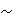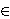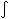Students / SubjectsHandbook > Decision-Making Under Uncertainty > Advanced Topics > Dual TheoryPrinter Friendly

Decision-Making Under Uncertainty - Advanced Topics

Yaari's Dual Theory

The dual theory, developed by Menahem Yaari, shifts the focus of a utility function for choices under uncertainty to being linear in probabilities and non-linear in payoffs -- as is expected utility -- to being non-linear in probabilities and linear in payoffs. The aim was to explain behavioral traits that are at odds with expected utility theory, and at this, it succeeds. However, it does create a number of anomalies of its own that are not present in expected utility, and in fact are the exact reverse. It remains important, though, as a vital step in the development of theories of choice under uncertainty that are tractable, have straightforward applications, and none of the behavioral irregularities of standard expected utility theory.

Probability Representation

To begin with, Yaari defines a decumulative distribution function (DDF) of a random variable v, representing a lottery, as:
Gv = Pr{v > t}, 0t1
So that integrating Gv(t)dt over the [0,1] interval gives the expected value of v. Restricting the value of v to the unit interval implies that no gambles can be made which might result in a loss greater than one's total wealth, & that no gambles exist offering prizes larger than a predetermined number. The gambles are all then normalized.

The Axioms

1. Neutrality: If u & v are two lotteries, then Gu = Gv implies uv.
2. Complete weak order: The preference relationis reflexive, transitive, and connected.
3. Continuity: For all DDFs G, G', H, H', such that G > G', there exists> 0 such that |G - H| <& |G' - H'| <imply H > H', where | | is the L1-norm, i.e. |m| is the integral of m(t)dt.
4. Monotonicity: With respect to first-order stochastic dominance, if Gu(t)Gv(t), then Gu(t)Gv(t).

Using these 4 axioms, we have an independence axiom:

5. Dual Independence: Instead of postulating independence for convex combinations formed along the probability axis, we postulate independence for convex combinations formed along the payments axis. This is done by specifying inverses of distribution functions as under:

G^(t) = {x|G(t)xG(t-)}, where G(t-) is the limit of G(s) as s tends to t from below, for t > 0. G(0-) = 1. We define the inverse of G as:

G-1(p) = min{t|pG^(t)}, & (G-1)-1 = G.

For a such that 0a1, & DDFs G, G', & H, we have a mixture operation such that:

aG(1-a)H = (aG-1 + (1-a)H-1)-1

and we now define the dual independence axiom as: GG' implies aG(1-a)HaG'(1-a)H.

The representation theorem

A preference relationsatisfies axioms 1-5 if & only if there exists a continuous & nondecreasing real function f, defined on the unit interval, such that for all u & v:

uv01f(Gu(t))dt01f(Gv(t))

References

Yaari, M. (1987) "The Dual Theory of Choice Under Risk." Econometrica. vol. 55 no. 1, pg 95-115.